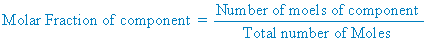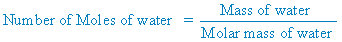Click to Chat

1800-1023-196

+91-120-4616500

CART 0

• 0

MY CART (5)

Use Coupon: CART20 and get 20% off on all online Study Material

ITEM
DETAILS
MRP
DISCOUNT
FINAL PRICE
Total Price: Rs.

There are no items in this cart.
Continue Shopping
`        The vapour pressure of water is 12.3 kpa at 300k. calculate the vapour pressure of 1 molal solution of a non volatile  solute in it? `
2 years ago

```							Step-1 what is given in problemVapour pressure of pure water = 12.3 kPaWe have 1 molal solution that means we have 1 moles of solute in 1kg of solventStep – 2 Find vapour pressureThe formula of vapour pressure when non-volatile liquid is addedPressure = vapour pressure of pure liquid × molar fraction of liquidP = P(pure) × X                                                                                  ... (1)Here we know the value of P(pure)so need to find the value of molar fraction X... (2)Here we know that number of moles of solute = 1 molesAnd need to find the moles of solvent (water)...(3)And we have mass = 1 kg and 1 kg is equal to 1000gAnd molar mass of water H2O = 2×1 + 16 = 18 g/molPlug the value in equation (3) we getNumber of moles of water =1000 g / (18g/mol  )  =55.56 molesPlug the value in equation (2) we getThe formula of molar fraction =55.5/(55.5+1)  = 0.9823Now plug in equation (1 )we getPartial fraction = 12.3×0.9823 = 12.08
```
2 years ago
Think You Can Provide A Better Answer ?

## Other Related Questions on Organic Chemistry

View all Questions »### Course Features

• 731 Video Lectures
• Revision Notes
• Previous Year Papers
• Mind Map
• Study Planner
• NCERT Solutions
• Discussion Forum
• Test paper with Video Solution### Course Features

• 70 Video Lectures
• Revision Notes
• Test paper with Video Solution
• Mind Map
• Study Planner
• NCERT Solutions
• Discussion Forum
• Previous Year Exam Questions